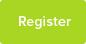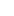# Tutor Hunt Questions

How do I predict the outcome of a reaction and its energetic driving force from the reactants alone? The notes and lecture slides don`t mention any of this but it is in an exam past paper.

One question is BF3 + O(C2H5)2
3 years ago

Please enter your response to the question below. The student will get a notification as soon your response has been approved by our moderation team.

Any chemical reaction has two aspects to bear in mind- rate (kinetics) and the equilibrium state to which the system goes.

A reaction can be feasible thermodynamically but very slow kinetically eg. Glass turning into Quartz.

The thermodynamics equation in the syllabus is : deltaG = deltaH - T*deltaS

If deltaG is negative then the reaction is feasible. Even this does not describe quite a few reactions.j

From the reagents alone without the above information you cannot predict it.
Remember Standard Reduction Potentials are a function of deltaG also.

Beyond this I would need to see the full question to comment further.

I hope this helps you.
Hi
This type of question is about bond energies. There should be a table of bond energies, either in the question or on a data sheet.
What you do is
1. count up the number of each type of bond in the reactants (ie 1 x C-C bonds, 3 x C-H bonds, 1 x O=O bonds) and calculate how much energy is needed to break those bonds.
2. now count up the number of each type of bond in the products and calculate how much energy is produced when the bonds form. You use the same table. When those bonds are formed they produce the same energy out as the energy needed to break them
NOTE: breaking bonds uses energy. Making bonds is the opposite, so it produces (gives out) energy.
3. The energy you put IN to break the bonds in the reactants is POSITIVE, the energy coming out as bonds form in the products is NEGATIVE (it comes out of the chemicals).
4. Put the 2 numbers together.
If the energy IN to break the bonds is bigger than the energy OUT when bonds form then chemicals have taken in energy overall so it is ENDOTHERMIC
If the energy OUT from creating bonds in the products is bigger than the energy IN to break the bonds then the chemicals have lost energy, so it is EXOTHERMIC (energy - usually as heat) goes out into the environment.

I hope this helps.